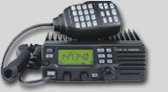Amateur Vertical Antenna Calculator

Here is a simple Javascript calculator for determining the lengths of tubing to be used for the construction of a Super J-Pole Collinear Design. (Note: It is always good practice to use an Antenna Analyzer when building a custom antenna!)

 Designation Number Required Data Entry Desired Frequency Mhz Select Antenna 1/8 1/4 3/8 1/2 5/8 3/4 7/8 Full Wavelength Calculated Results Calculated Vertical Length

This calculator is designed to give the vertical length (height) of a particular antenna, for the frequency and wavelength chosen. This does NOT take into consideration the type or size of horizontal reflectors, of even if there is a need for them. Enter the desired frequency and select the wavelength. Click on Calculate and the optimum length for that combination will be displayed in feet, inches and fractions of inches, and in meters. To optimize the antenna for a frequency RANGE, do the calculations twice, once for the low end of the range and once for the high end; then average the two and plan to adjust the VSWR on both ends of the range as needed.Popular Callsign Lookups
for this month:

K5IZ
N8BNC
KD8GKJ

N2OXR